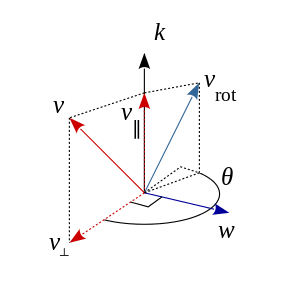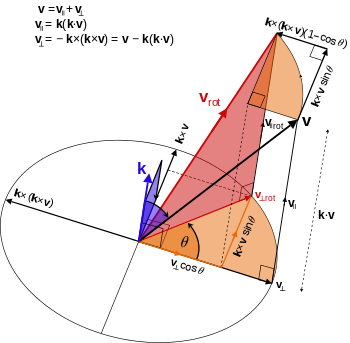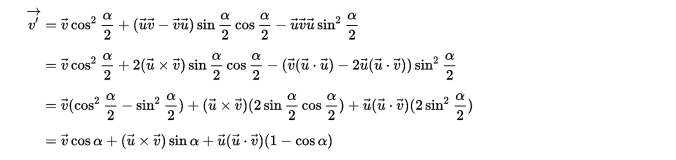# 四元数与旋转变换数学 同时被 2 个专栏收录3 篇文章 0 订阅
1. 问题来源
问题还是来源于课本内容，在图形学课本中讲到三维变换矩阵的时候引入了四元数，但是同样没有说明四元素是如何旋转三维空间里向量的原因。通过查找维基百科，这个问题完全可以理解。
首先简单介绍四元数的表示形式：

q=s+xi+yj+zk   s,x,y,zR

其中i,j,k定义它们的计算规则是 i2=j2=k2=ijk=1 $\mathbf i^{2} = \mathbf j^{2} = \mathbf k^{2} = \mathbf i\mathbf j\mathbf k = -1$ 以及:
ij=k   jk=i   ki=j
ji=k   kj=i   ik=j
s是实部， i $\mathbf i$, j $\mathbf j$, k $\mathbf k$是虚数的单位，进行乘运算时又有类似向量叉积的特点，不可以交换计算顺序，关于四元数介绍和运算可以看这里。把这三个分量看成是三位空间向量的坐标，则两个纯四元数(实部s=0) u $\overrightarrow u$ v $\overrightarrow v$之间的乘积可以用向量的运算来表示： uv=u×vuv(1.1) $\overrightarrow u\overrightarrow v=\overrightarrow u\times \overrightarrow v-\overrightarrow u\cdot \overrightarrow v \quad (1.1)$注意式子中，在讨论纯四元数的时候，对四元数和向量的表示并没有区分开来，后面也不加区分的使用这种表示。

2. 四元数与万向节死锁的关系
关于万向节死锁的有很多形象的例子和解释，例如[http://www.cnblogs.com/soroman/archive/2006/10/11/526163.html]（请注意例子中所使用的坐标系）。在这里必须澄清和强调一个事实，望远镜在竖直指向天空(笛卡儿坐标系z坐标轴方向)的情况下，无法追踪突然转向南方飞行的飞行器的原因，并非是望远镜不能够指向飞行器，而是望远镜无法依赖转向轴的旋转而指向飞行器。归根结底在于望远镜只能依赖x,y两个转向轴进行旋转（请注意这种的表述）,想要以x轴旋转改变向量的x坐标不可能。意思是在三维空间的笛卡儿坐标系中，仅仅依赖x,y,z轴并不能旋转某些向量的方向，而所有按欧拉角旋转的矩阵使用的旋转轴是xyz轴。过分理解万向节死锁的原因不可取。个人看法：在绕xyz轴旋转无法旋转目标向量方向时，最多能够引出该绕什么轴旋转的问题。四元数运用的三维空间的变换，跟万向节死锁现象没有太明显的关系，因为无论绕什么轴旋转最终都能转换到绕xyz轴旋转。那么，如何在三维空间中表示向量绕任意轴旋转的公式？

3. 罗德里格斯旋转公式(Rodrigues’rotation formula)
罗德里格斯旋转公式，运用向量的点积和叉积的运算，让人大开眼界，又一次填充了我的数学空白。向量 v $\mathbf v$绕单位向量 k $\mathbf k$旋转 θ $\theta$ 角度后变为向量 vrot $\mathbf v_{rot}$。向量 v $\mathbf v$可以分解成垂直于和平行于单位向量 k $\mathbf k$的两个分量 v $v_{\bot}$以及 v $v_{\|}$，由于无法避开数学公式推导，所以下面的公式推导完全是拷贝的维基百科上的内容
v=v+v(1) $v = v_{\bot} + v_{\|} \quad (1)$同样旋转后的 vrot=vrot+v $v_{rot} = v_{\bot rot} + v_{\|}$其中平行的分量保持不变，只有垂直的分量 v $\mathbf v_{\bot}$在旋转中发生改变为 vrot $\mathbf v_{\bot rot}$。见下图图中的标注(注意坐标轴的方向与计算过程)得出。
vrot=cosθv+sinθk×v $v_{\bot rot} = \cos\theta v_{\bot}+\sin\theta k\times v$
最终：
vrot=v+cosθv+sinθk×v=v+cosθ(vv)+sinθk×v=cosθv+(1cosθ)v+sinθk×v=cosθv+(1cosθ)(kv)k+sinθk×v

这个等式表示了向量 v $\mathbf v$绕向量 k $\mathbf k$旋转后的结果，虽然这个式子看上去比较简洁，实际上在向量空间中把旋转后的结果 vrot $\mathbf v_{rot}$的坐标表示出来，还要计算点积和叉积的结果。个人看法：向量的点积和叉积引入公式之后，目的正是为了简化了很大计算量的算式表示形式。还有一些叉积的运算公式，也很巧妙，有兴趣可以参上面的维基链接地址，下文将会引用到这些计算公式。
4. 四元数与空间旋转变换[Quaternions ]
如果空间向量 v $\overrightarrow v$想绕某一个单位向量 u $\overrightarrow u$旋转 α $\alpha$度角，则使用四元数 q=cosα2+usinα2 $q=\cos\frac{\alpha}{2}+\overrightarrow u\sin\frac{\alpha}{2}$以及它的共轭四元数与 v $\overrightarrow v$进行汉密尔顿积得到的旋转后的新向量:
v=qvq1=(cosα2+usinα2)v(cosα2usinα2)

具体证明见：u×(v×u)=(uu)v(uv)u

uvu=(u×vuv)u=(u×v)×u(u×v)u(uv)u=u×(v×u)(uv)u=(uu)v2(uv)u

http://www.qiujiawei.com/understanding-quaternions/
https://en.wikipedia.org/wiki/Quaternions_and_spatial_rotation
https://en.wikipedia.org/wiki/Triple_product#Vector_triple_product

09-1808-22
04-252万+
11-236758
03-2369
06-014259
06-017851
05-202万+
11-18838
07-12585
10-26885
03-291万+
08-1786
06-011万+
02-123万+
07-051万+点击重新获取扫码支付余额充值